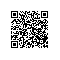# 使用Python和Perl绘制北京跑步地图

（本文工程已经托管在Github,https://github.com/ferventdesert/gpx-crawler）

## 1.数据来源：益动GPShttp://edooon.com/user/5699607196/record/15414378## 2. 获取益动网站上的数据folder = u'D:/buptzym的同步盘/百度云/我的文档/数据分析/datasets/rungps/';
cookie='JSESSIONID=69DF607B71B1F14AFEC090F520B14B55; logincookie=5699607196$6098898D08E533587E82B33DD9D02196; persistent_cookie=5699607196$42C885AD38F59DCA407E09C95BE1A60B; uname_forloginform="buptzym@qq.com"; __utma=54733311.82935663.1447906150.1447937410.1456907433.7; __utmb=54733311.5.10.1456907433; __utmc=54733311; __utmz=54733311.1456907433.7.3.utmcsr=baidu|utmccn=(organic)|utmcmd=organic; cookie_site=auto'
userid='5699607196';
f = codecs.open(folder + 'desert.htm', 'r', 'utf-8');
f.close();
root = etree.HTML(html)
tree = etree.ElementTree(root);

listnode=tree.xpath('//*[@id="feedList"]');
numre=re.compile(u'骑行|跑步|公里|，|耗时|消耗|大卡');
urllists=[]
records=[];
for child in listnode.iterchildren():
record={};
temp=child.xpath('div/div/a')
if len(temp)==0:
continue;
source= temp.attrib['href'];
record['id']=source.split('/')[-1];
info=temp.text;
numinfo= numre.split(info);
if len(numinfo)<6:
continue;
record['type']= info[0:2];
record['distance']= numinfo;
record['hot']=numinfo;
urllists.append('http://edooon.com/user/%s/record/export?type=gpx&id=%s' % (userid, record['id']));

opener = urllib.request.build_opener()
path='//*[@id="exportList"]/li/a';
for everyURL in urllists:
id = everyURL.split('=')[-1];
print(id);
url='http://edooon.com/user/%s/record/%s' % (userid, id);
f = opener.open(url);
f.close();
root = etree.HTML(html)
tree = etree.ElementTree(root);
fs = str(tree.xpath(path));
if fs is None:
continue;
furl = 'http://edooon.com/user/%s/record/%s' % (userid, fs);
f = opener.open(furl);
f.close();
filename=folder+'id'+'.gpx';
xmlfile = codecs.open(filename, 'wb');
xmlfile.write(html);
xmlfile.close();

## 3. 解析gpx数据

pip3 install gpxpy 即可安装。

gpxpy提供了丰富的接口，当然为了统计，我们只需要提取一部分数据：

def readgpx(x):

file= open(dir+x+'.gpx','r')
gpx=gpxpy.parse(txt)
mv=gpx.get_moving_data()
dat= {'移动时间':mv.moving_time,'静止时间':mv.stopped_time,'移动距离':mv.moving_distance,'暂停距离':mv.stopped_distance,'最大速度':mv.max_speed};
dat['总时间']=(gpx.get_duration())
dat['id']=str(x)
updown=gpx.get_uphill_downhill()
dat['上山']=(updown.uphill);
dat['下山']=(updown.downhill)
timebound=gpx.get_time_bounds();
dat['开始时间']=(timebound.start_time)
dat['结束时间']=(timebound.end_time)
p=gpx.get_points_data()
dat['lat']=p.point.latitude
dat['lng']=p.point.longitude
file.close()
return datexceptids=详细[(详细.lng<116.1)|(详细.lng>116.7)|(详细.lat<39.9)|(详细.lat>40.1)].id

def filtercity(r):
sp=r.split('/')[-1].split('.')
if sp!='gpx':
return False;
if sp in exceptids.values:
return False;
return True;

bjids= [r for r in gpxs if filtercity(r)]


# 4.绘制GPS数据

Ubuntu默认安装Perl, GD是需要libgd的，libgd却在官网上极难下载，下载后却又发现版本不对，这让我在国外互联网上遨游了好几个小时，都要死掉了。。。到最后，我才发现，安装libgd库只要下面这一步就可以了：

apt-get install libgd-gd2-perl

perl ./Makefile.PL

make

make install

This folder contains several Perl scripts for processing and plottin  GPS track data in .GPX format.

./runme.sh



## 5.总结

GD还需要libpng等一众perl库，在附件里都有提供下载。

#### 附件是GD库和爬取所有gpx数据的python3代码。使用钉钉扫一扫加入圈子
+ 订阅